>

## Chapter 4 triangles R.D. Sharma Solutions for Class 10th Math Exercise 4.2

Exercise 4.2

1. In ΔABC, D and E are points on the sides AB and AC respectively such that DE | | BC

(i) AD = 6 cm, DB = 9 cm and AE = 8 cm, find AC.
(ii) If AD/DB = 3/4 and AC = 15 cm, find AE
(iii) If AD/DB = 2/3 and AC = 18 cm, find AE
(iv) If AD = 4, AE = 8, DB = x-4, and EC = 3x – 19, find x.
(v) If AD = 8 cm, AB = 12 cm and AE = 12 cm, find CE.
(vi) If AD = 4 cm, DB = 4.5 cm and AE = 8 cm, find AC .
(vii) If AD = 2 cm, AB = 6 cm and AC = 9 cm, find AE.
(viii) If AD/BD = 4/5 and EC = 2.5 cm, find AE .
(ix) If AD = x, DB = x – 2, AE = x+2 and EC = x – 1, find the value of x.
(x) If AD = 8x – 7, DB = 5x – 3, AE = 4x – 3 and EC = (3x -1), find the value of x.
(xi) If AD = 4x – 3, AE = 8x – 7, BD = 3x – 1 and CE = 5x – 3, find the value of x.
(xii) If AD = 2.5 cm, BD = 3.0 cm and AE = 3.75 cm, find the length of AC .

Solution

(i)(ii)(iii)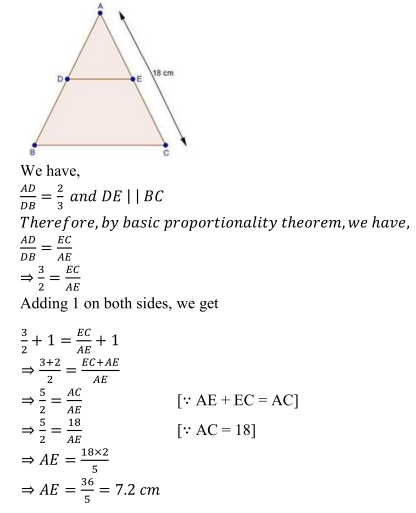(iv)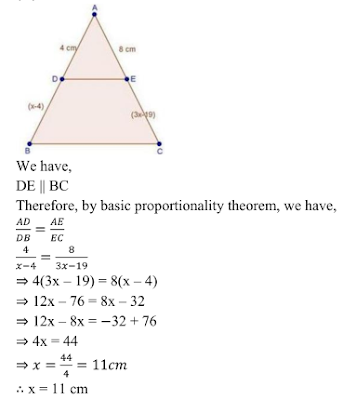(v)(vi)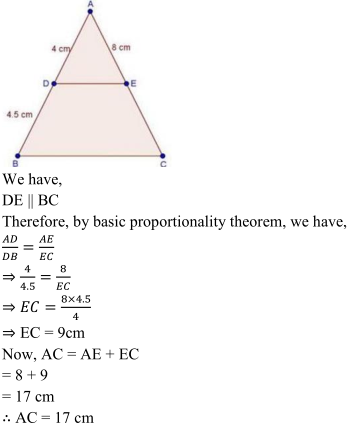(vii)(viii)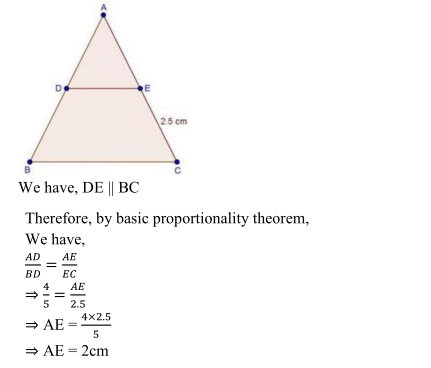(ix)(x)(xi)(xii)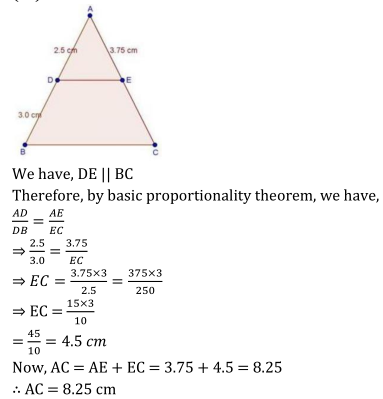2. In a ∆ABC, D and E are points on the sides AB and AC respectively. For each of the
following cases show that DE | | BC:
(i) AB = 2cm, AD = 8cm, AE = 12 cm and AC = l8cm.
(ii) AB = 5.6cm, AD = 1.4cm, AC = 7.2 cm and AE = 1.8 cm.
(iii) AB = 10.8 cm, BD = 4.5 cm, AC = 4.8 cm and AE = 2.8 cm.
(iv) AD = 5.7 cm, BD = 9.5 cm, AE = 3.3 cm and EC = 5.5 cm.

Solution
(i)(ii)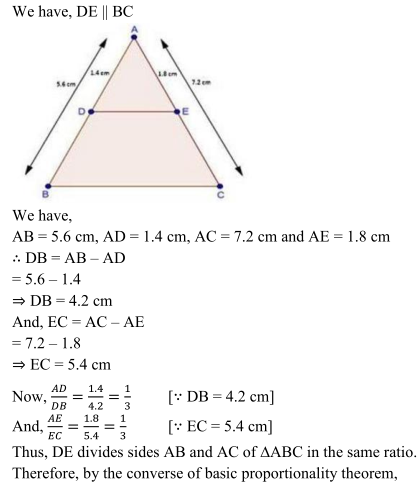(iii)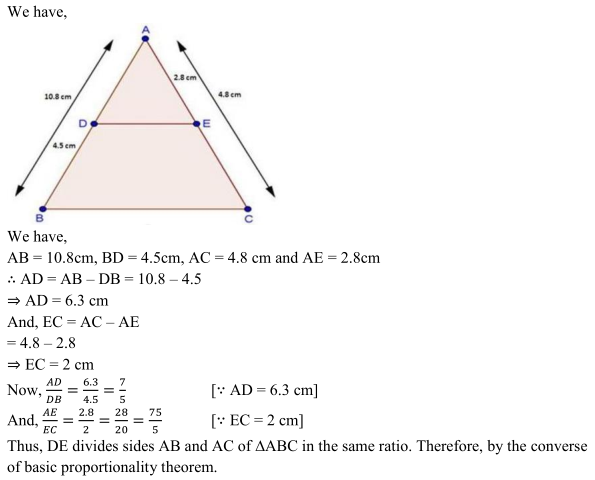(iv)3. In a ∆ABC, P and Q are points on sides AB and AC respectively, such that PQ || BC. If AP= 2.4 cm, AQ = 2 cm, QC = 3 cm and BC = 6 cm, find AB and PQ.

Solution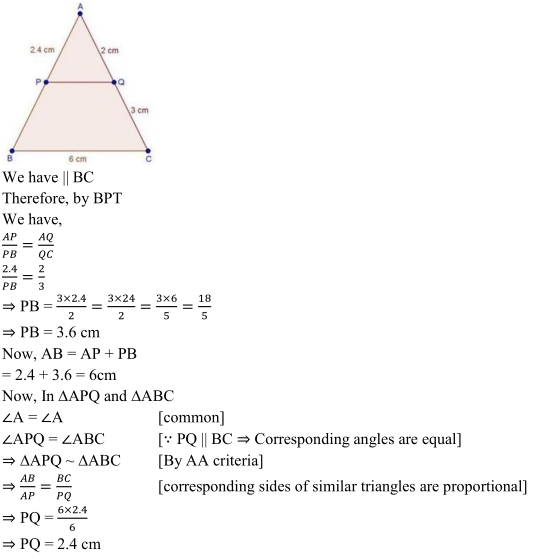4. In a ∆ABC, D and E are points on AB and AC respectively such that DE || BC. If AD = 2.4cm, AE = 3.2 cm, DE = 2cm and BC = 5 cm, find BD and CE.
Solution5. In below Fig., state if PQ || EF.Solution6. M and N are points on the sides PQ and PR respectively of a ∆PQR. For each of the following cases, state whether MN || QR
(i) PM = 4cm, QM = 4.5 cm, PN = 4 cm and NR = 4.5 cm
(ii) PQ = 1.28 cm, PR = 2.56 cm, PM = 0.16 cm, PN = 0.32

Solution
(i)(ii) For MN | | QR, by Theles theorem the required condition is :
PM/PQ = PN/PR
PM/PQ = 0.16/1.28 = (16/100)/(128/100)
= 16/128 = 1/8

PN/PR = 0.32/2.56 = 32/256 = 1/8
PM/PQ = PN/PR
Therefore MN | | QR.

7. In three line segments OA, OB, and OC, points L, M, N respectively are so chosen that LM || AB and MN || BC but neither of L, M, N nor of A, B, C are collinear. Show that LN || AC.
Solution8. If D and E are points on sides AB and AC respectively of a ∆ABC such that DE || BC and BD = CE. Prove that ∆ABC is isosceles.

Solution